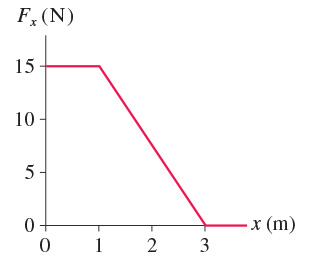Problem: A 400 g particle moving along the x-axis experiences the force shown in the figure. The particle's velocity is 9.0 m/s at x = 0 m. What is its velocity at x = 3 m?

95% (75 ratings)
Problem Details
A 400 g particle moving along the x-axis experiences the force shown in the figure. The particle's velocity is 9.0 m/s at x = 0 m. What is its velocity at x = 3 m?